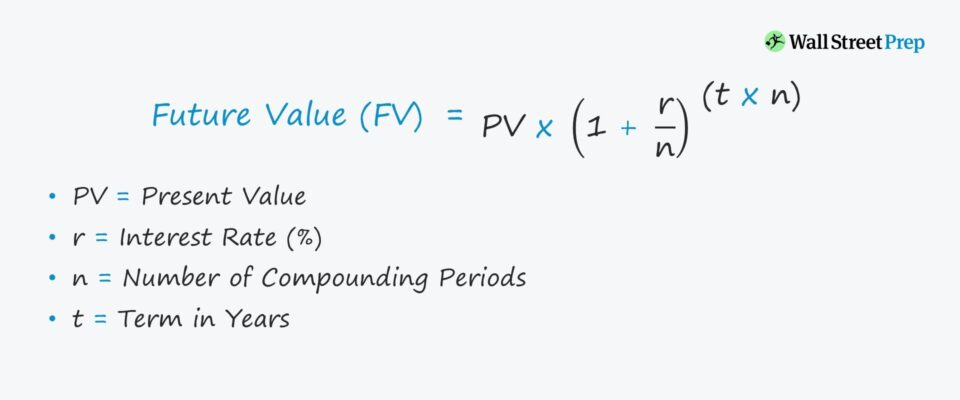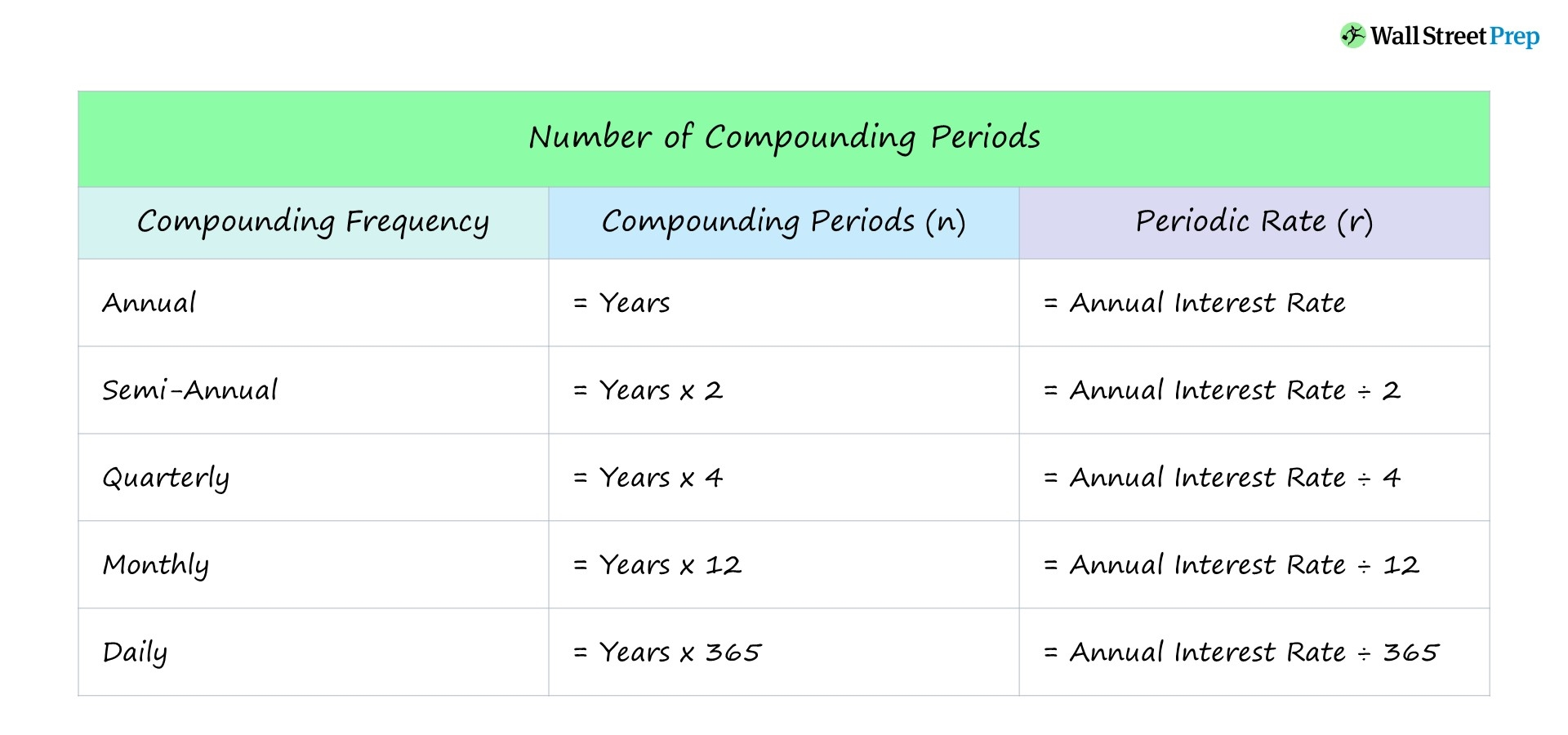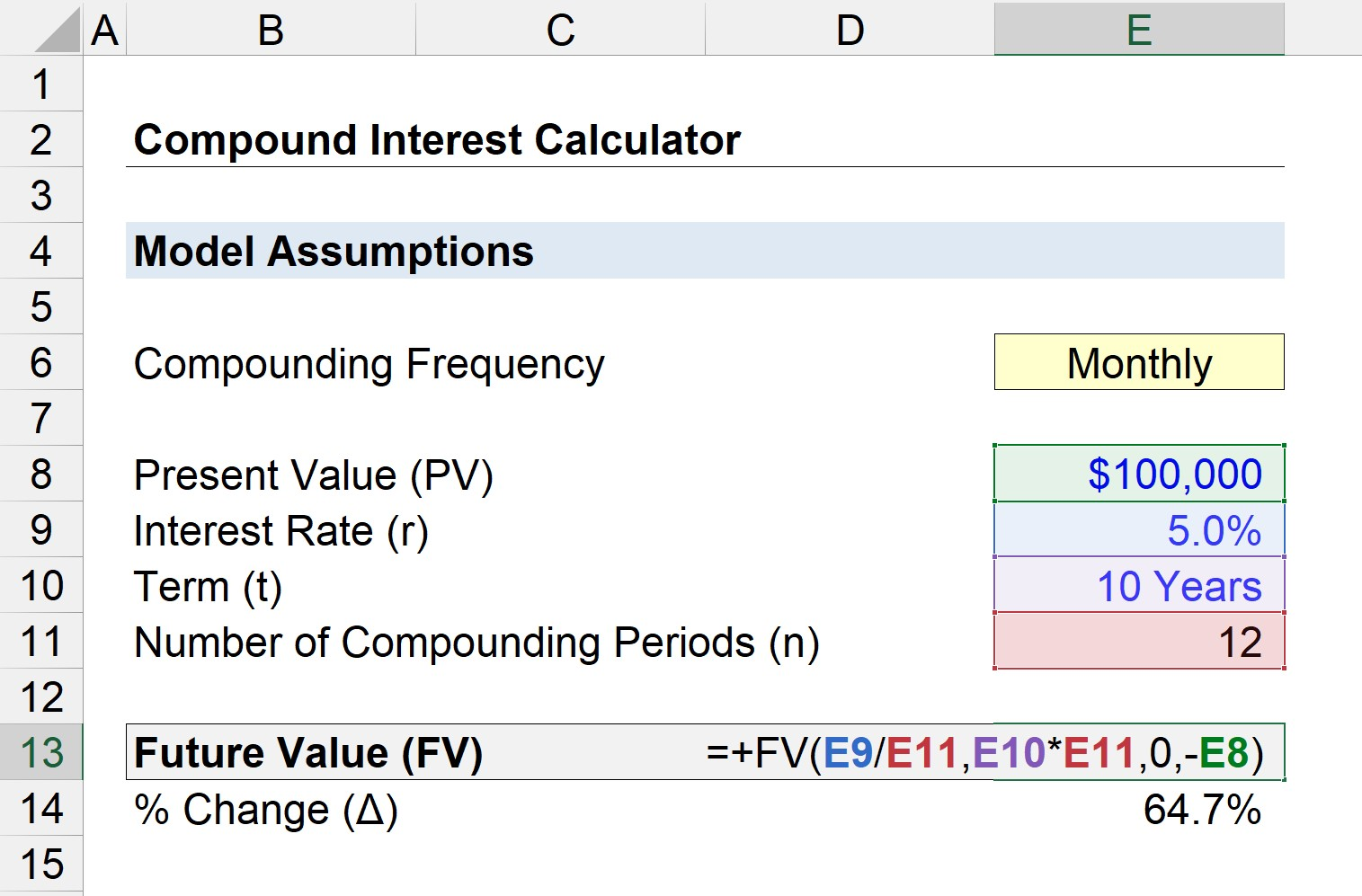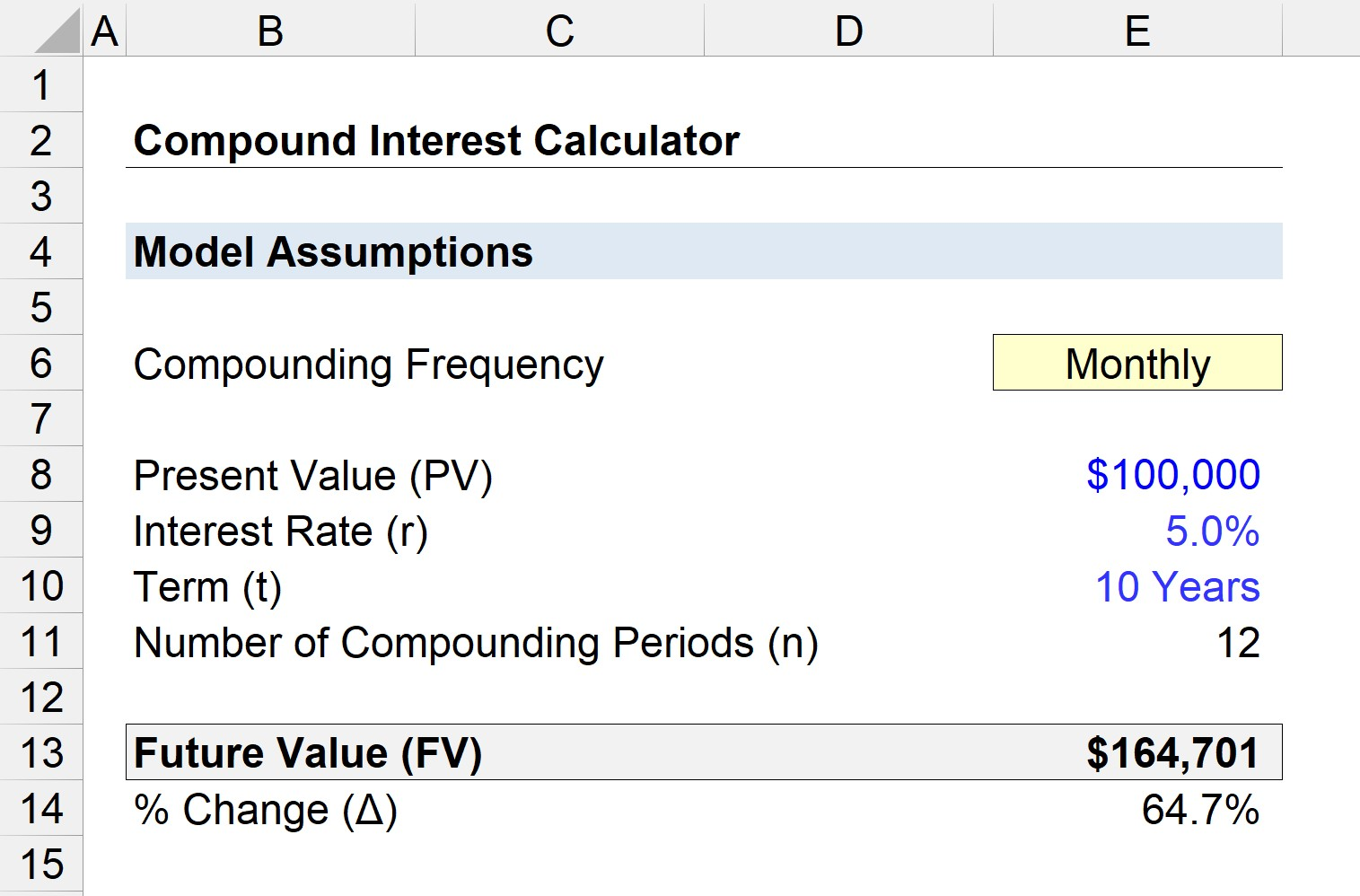Welcome to Wall Street Prep! Use code at checkout for 15% off.# Compound Interest

Guide to Understanding Compound Interest## How to Calculate Compound Interest?

In finance, compound interest stems from growth in the principal amount from the accumulation of interest, resulting in more interest being received (i.e. “interest on interest”).

Conceptually, the notion of compound interest can be described as earning “interest on interest.”

Here, interest is earned on two components:

1. Original Principal: Initial Amount Invested, Borrowed, or Lent
2. Accumulated Interest: Interest from Earlier Periods (i.e. “Interest on Interest”)

The accumulated interest is added to the principal amount, which subsequently determines the interest amount in the next period in a continuous cycle until the end of the term.

Therefore, even with a low-interest rate, the effects of compounding can cause the principal to grow substantially over a long time horizon.

## Compound Interest Chart

Compounding is a central piece of the decision-making process by investors, borrowers, and lenders.

The rate at which the compounding effects on interest accumulate is a function of the frequency of compounding periods.

The greater the number of compounding periods, the greater the effects (i.e. the “snowball effect”).## Compound Interest Formula

The formula for calculating the future value of an interest-earning financial instrument with the effects of compounding is shown below:

Future Value (FV) = PV [1 + (r ÷ n)] ^ (n × t)

Where:

• PV = Present Value
• r = Interest Rate (%)
• t = Term in Years
• n = Number of Compounding Periods

The number of compounding periods is equal to the term in years multiplied by the corresponding factor.

• Daily Compounding: 365x Per Year
• Monthly Compounding: 12x Per Year
• Quarterly Compounding: 4x Per Year
• Semi-Annual Compounding: 2x Per Year
• Annual Compounding: 1x Per Year

If we subtract the present value (PV) from the future value (FV), the impact of compounding interest can be isolated.

## Compound Interest vs. Simple Interest: What is the Difference?

Unlike simple interest, “compound” interest is based on the principal amount plus any accrued interest.

In each compounding period, the interest accrued in the previous period is rolled-forward into the current period and increases the principal amount.

By contrast, the accumulated interest is not added to the principal in simple interest calculations. Instead, simple interest is calculated off of the original principal amount.

Simple Interest = PV × r × t

Where:

• PV = Present Value
• r = Interest Rate (%)
• t = Term in Years

## PIK Interest Concept: Accrued Interest Expense

PIK interest, or “paid in kind” interest, is another variation to be aware of.

Here, the interest expense accrues to the ending principal, rather than being paid out in cash in the current period.

But while the borrower is able to delay the owed payment, the effects of compounding cause the principal balance that must be paid at the date of maturity to increase in value.

## Compound Interest Rate Calculator

We’ll now move to a modeling exercise, which you can access by filling out the form below.Submitting...

## 1. Compound Interest Rate Assumptions

Suppose you’ve decided to deposit \$100,000 into a bank account.

If we assume the annual interest rate (r) is 5% and the deposit was left untouched for 10 years, how much the original \$100,000 is worth in the future is determined by the compounding frequency.

• Interest Rate (r) = 5%
• Present Value (PV) = \$100,000
• Term (t) = 10 Years

## 2. Future Value Calculation (FV Excel Function)

The “FV” Excel function can be used to calculate how much your \$100,000 deposit is now worth after 10 years.

=FV (rate, nper, pmt, pv)

Where:

• rate = Interest Rate (%)
• nper = Term in Years x Number of Compounding Periods
• pmt = 0
• pv = – Present Value (Principal)

Since the \$100,000 was an outflow from your perspective (i.e. an investment), it should be entered as a negative figure.## 3. Compound Interest Rate Calculation and Returns Analysis

Under each scenario, the future value (FV) of the \$100,000 deposit and the percentage change compared to the original value is shown below:

• Annual Compounding: \$162,899 (62.9%)
• Semi-Annual Compounding: \$163,862 (63.9%)
• Quarterly Compounding: \$164,362 (64.4%)
• Monthly Compounding: \$164,701 (64.7%)
• Daily Compounding: \$164,866 (64.9%)

The deposit earns the difference between the future value (FV) and the present value (PV).

• Annual: \$162,899 – \$100,000 = \$62,899
• Semi-Annual: \$163,862 – \$100,000 = \$63,862
• Quarterly: \$164,362 – \$100,000 = \$64,362
• Monthly: \$164,701 – \$100,000 = \$64,701
• Daily: \$164,866 – \$100,000 = \$64,866

For instance, if the compounding frequency is monthly, your \$100,000 deposit has grown to \$164,701, netting a total of \$64,701 in interest after 10 years.

To reiterate from earlier, the more frequently that interest is compounded, the more interest is earned, as our compound interest model confirms.Step-by-Step Online Course

### Everything You Need To Master Financial Modeling

Enroll in The Premium Package: Learn Financial Statement Modeling, DCF, M&A, LBO and Comps. The same training program used at top investment banks.Inline FeedbacksSharron Dupree
April 24, 2023 12:09 am

Excellent information. Thank you.April 24, 2023 8:54 am

You’re welcome, Sharron!Learn Financial Modeling Online

Everything you need to master financial and valuation modeling: 3-Statement Modeling, DCF, Comps, M&A and LBO.

X

The Wall Street Prep Quicklesson Series

7 Free Financial Modeling Lessons

Get instant access to video lessons taught by experienced investment bankers. Learn financial statement modeling, DCF, M&A, LBO, Comps and Excel shortcuts.